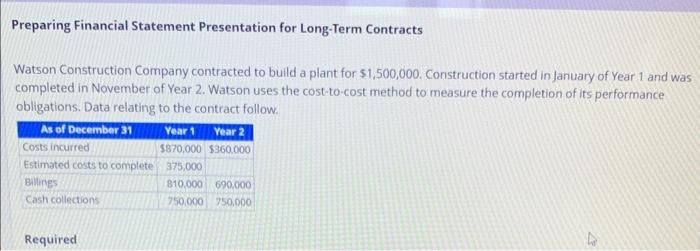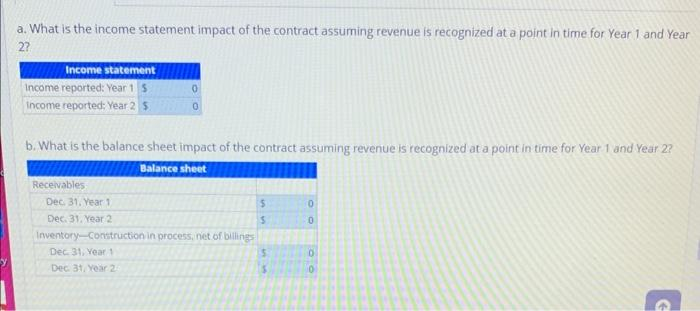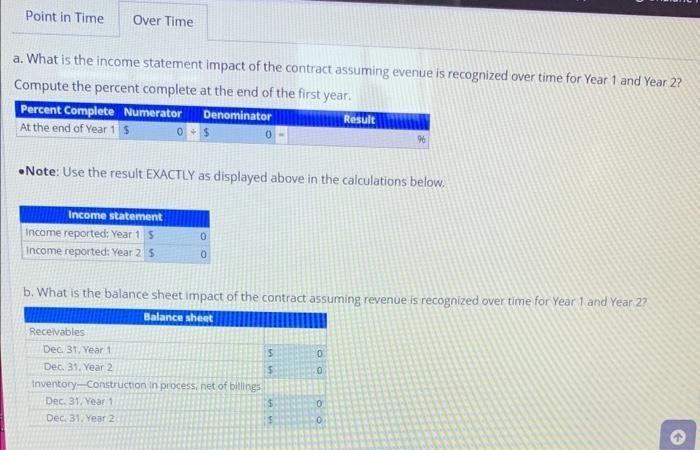# Question Preparing Financial Statement Presentation for Long-Term Contracts Watson Construction Company contracted to build a plant for $$\ 1,500,000$$. Construction started in January of Year 1 and was completed in November of Year 2. Watson uses the cost-to-cost method to measure the completion of its performance obligations. Data relating to the contract follow. Required a. What is the income statement impact of the contract assuming revenue is recognized at a point in time for Year 1 and Year 2? b. What is the balance sheet impact of the contract assuming revenue is recognized at a point in time for Year 1 and Year 2 ? a. What is the income statement impact of the contract assuming evenue is recognized over time for Year 1 and Year 2 ? Compute the percent complete at the end of the first year. - Note: Use the result EXACTLY as displayed above in the calculations below. b. What is the balance sheet impact of the contract assuming revenue is recognized over time for Year 1 and Year 2 ?Transcribed Image Text: Preparing Financial Statement Presentation for Long-Term Contracts Watson Construction Company contracted to build a plant for $$\ 1,500,000$$. Construction started in January of Year 1 and was completed in November of Year 2. Watson uses the cost-to-cost method to measure the completion of its performance obligations. Data relating to the contract follow. Required a. What is the income statement impact of the contract assuming revenue is recognized at a point in time for Year 1 and Year 2? b. What is the balance sheet impact of the contract assuming revenue is recognized at a point in time for Year 1 and Year 2 ? a. What is the income statement impact of the contract assuming evenue is recognized over time for Year 1 and Year 2 ? Compute the percent complete at the end of the first year. - Note: Use the result EXACTLY as displayed above in the calculations below. b. What is the balance sheet impact of the contract assuming revenue is recognized over time for Year 1 and Year 2 ?
Transcribed Image Text: Preparing Financial Statement Presentation for Long-Term Contracts Watson Construction Company contracted to build a plant for $$\ 1,500,000$$. Construction started in January of Year 1 and was completed in November of Year 2. Watson uses the cost-to-cost method to measure the completion of its performance obligations. Data relating to the contract follow. Required a. What is the income statement impact of the contract assuming revenue is recognized at a point in time for Year 1 and Year 2? b. What is the balance sheet impact of the contract assuming revenue is recognized at a point in time for Year 1 and Year 2 ? a. What is the income statement impact of the contract assuming evenue is recognized over time for Year 1 and Year 2 ? Compute the percent complete at the end of the first year. - Note: Use the result EXACTLY as displayed above in the calculations below. b. What is the balance sheet impact of the contract assuming revenue is recognized over time for Year 1 and Year 2 ?&#12304;General guidance&#12305;The answer provided below has been developed in a clear step by step manner.Step1/2Please refer to the excel spreadsheet for all the answers.ABCDEFGH1GivenAnswer a2Plant$1,500,0003As of December 31st12Income Reported : Year 1$ 180,000=B174Cost Incurred$870,000$360,000Income Reported : Year 2$90,000=C175Estimated cost to completion$375,0006Billings$810,000$690,000Receivables7Cash Collections$750,000$750,000Dec 31, year 1$810,000=B68Dec 31, year 2$ 690,000=C69Attributes to financial presentation Inventory-Construction in progress net of billings 1012Dec 31, year 1$60,000=B4-B611Costs Incurred to date$870,000$1,230,000=B11+C4Dec 31, year 2$ (330,000)=C4-C612Estimated additional costs$375,00013Estimated Total Costs$1,245,000\$1,230,000=sum(C11:C12)Answe ... See the full answer